## Consider an urn containing 4 white balls and 1 black ball, and suppose that balls will be sequentially drawn (without replacement) from the

Question

Consider an urn containing 4 white balls and 1 black ball, and suppose that balls will be sequentially drawn (without replacement) from the urn until the black ball is obtained. Letting X denote the number of white balls drawn before the black one is selected, give the pmf of X.

in progress 0
4 weeks 2021-08-18T03:27:17+00:00 1 Answers 0 views 0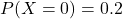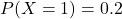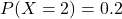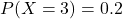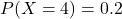Step-by-step explanation:

A probability is the number of desired outcomes divided by the number of total outcomes.

pmf of X.

Probability of each outcome, 0, 1, 2, 3 or 4 white balls drawn.

Before the trials, you are equally as likely to get the black ball in the 1st trial(0 white balls drawn) to the 5th trial(4 white balls drawn). So this is an uniform distribution with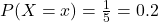Thus, the pmf is: